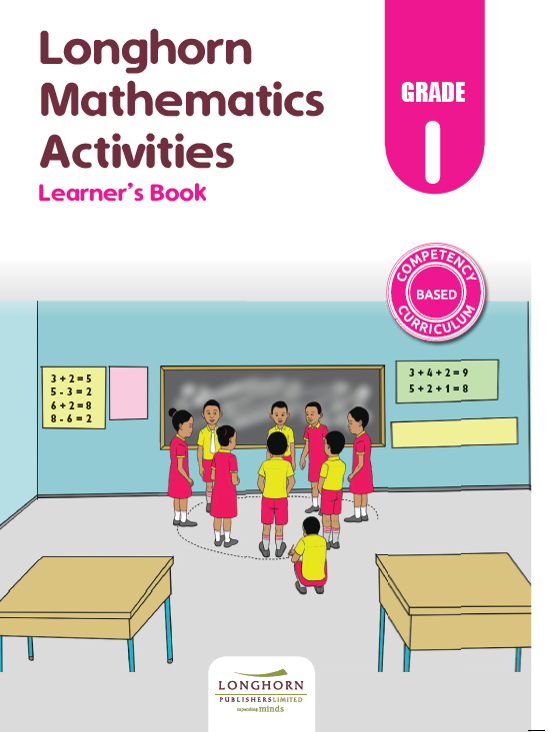Study kit (Math 1)##### Authors
T. Mukhuri, M. Okello, M. Mwimani, J. Mwaniki, S. Ndinwa
##### Exercises kit’s authors
T. Mukhuri, M. Okello, M. Mwimani, J. Mwaniki, S. Ndinwa
##### Publisher
Longhorn Publishers
##### Included in packages• The study kit contains 73 chapters and teacher’s exercises kit contains 182 exercises.
• ##### Authors
T. Mukhuri, M. Okello, M. Mwimani, J. Mwaniki, S. Ndinwa
• ##### Exercises kit’s authors
T. Mukhuri, M. Okello, M. Mwimani, J. Mwaniki, S. Ndinwa
Mathematics
English
• ##### Publisher
Longhorn Publishers

### 1. Number Concept

##### Chapter
1.1. Preparing ourselves
Free chapter!
1.2. Sorting and grouping
1.3. Matching same type of objects
1.4. Pairing objects of the same type
1.5. Comparing number of objects (1)
1.6. Comparing number of objects (2)
1.7. Comparing number of objects (3)
1.8. Order and sequence
1.9. Making patterns using real objects
1.10. Reciting number names up to 50
1.11. The count for a group

### 2. Whole numbers

##### Chapter
2.1. Counting up to 100 objects
2.2. Representing numbers from 1 to 50 using objects
2.3. Place value
2.4. Counting in tens and ones
2.5. Using abacii
2.6. Place value of ones and tens
2.7. Writing numbers 1 to 50 in symbols
2.8. Writing numbers 1 to 10 in words
2.9. Number patterns
2.10. Digital games

##### Chapter
3.2. Use of (+), (=) signs
3.3. Addition of 2 – single digit numbers
3.4. Addition using a family of 10
3.8. Addition of 3 single-digit numbers
3.10. Addition using the family of 10
3.11. Addition of 2-digit to 1-digit numbers without regrouping
3.13. Addition of multiples of 10
3.14. Number patterns

### 4. Subtraction

##### Chapter
4.1. Subtraction as taking away
4.2. Subtraction sentences using ‘–’ and ‘=’ signs
4.3. Subtraction of single-digit numbers
4.4. Vertical subtraction
4.5. Subtraction by counting backwards
4.6. Subtraction of up to 2 – digit numbers
4.7. Vertical subtraction without regrouping
4.8. Making subtraction sentences from addition sentences
4.9. Subtraction of multiples of 10
4.10. Number patterns

### 5. Length

##### Chapter
5.1. Comparing lengths of objects
5.2. Shorter than, longer than, same as
5.3. Conservation of length
5.4. Measuring length using arbitrary units

### 6. Mass

##### Chapter
6.1. Comparing masses of objects
6.2. Heavier than, lighter than
6.3. Same mass as
6.4. Conservation of mass
6.5. Measuring mass using arbitrary units

### 7. Capacity

##### Chapter
7.1. Comparing capacities of containers
7.2. Holds same as
7.3. Conservation of capacity

### 8. Time

##### Chapter
8.1. Daily activities related to time
8.2. Days of the week
8.3. Months of the year

### 9. Money

##### Chapter
9.1. Kenyan currency
9.3. Difference between needs and wants
9.4. Spending and saving

### 10. Geometry: Lines

##### Chapter
10.1. Straight lines
10.2. Curved lines
10.3. Curved and straight lines